Next: Open or stage-specified boundary Up: Specifying boundary conditions Previous: Specifying boundary conditions   Contents

### Open or velocity-specified boundary conditions

This file loops through the edges whose edge markers are 2. As an example, consider the flow in a channel that is 1000 m long in which two boundaries are specified as inflow and outflow and the other two are specified as solid walls, as shown in Figure 2.
 .5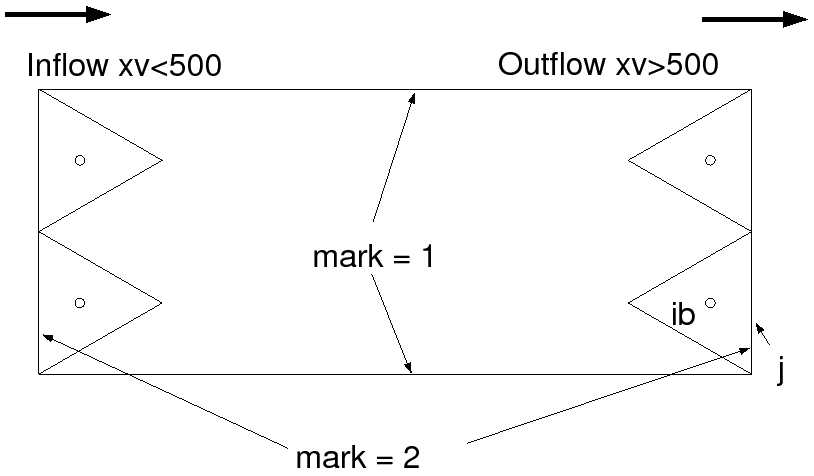Suppose you would like to specify an incoming velocity that oscillates at a frequency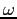due to an incoming wave at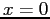m and that you would like to use the linearized open boundary condition to specify the outflow at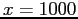m. Since the edge markers at the inflow and outflow are the same, you need to set the boundary condition based on the x,y location of the specified edge. To do so, you would set up the functions in the boundaries.c file as follows
• Function OpenBoundaryFluxes:
  for(jptr=grid->edgedist;jptr<grid->edgedist;jptr++) {
j = grid->edgep[jptr];

if(grid->xv[ib]>500) {
for(k=grid->etop[j];k<grid->Nke[j];k++)
ub[j][k] = -phys->h[ib]*sqrt(prop->grav/(grid->dv[ib]));
} else {
for(k=grid->etop[j];k<grid->Nke[j];k++)
ub[j][k]=phys->boundary_u[jptr-grid->edgedist][k]*grid->n1[j]+
phys->boundary_v[jptr-grid->edgedist][k]*grid->n2[j];
}
}

• BoundaryVelocities Function:
  for(jptr=grid->edgedist;jptr<grid->edgedist;jptr++)
j = grid->edgep[jptr];

if(grid->xv[ib]>500) {
for(k=grid->etop[j];k<grid->Nke[j];k++) {
phys->boundary_u[jptr-grid->edgedist][k]=phys->uc[ib][k];
phys->boundary_v[jptr-grid->edgedist][k]=phys->vc[ib][k];
phys->boundary_w[jptr-grid->edgedist][k]=
0.5*(phys->w[ib][k]+phys->w[ib][k+1]);
}
} else {
for(k=grid->etop[j];k<grid->Nke[j];k++) {
phys->boundary_u[jptr-grid->edgedist][k]=
prop->amp*cos(prop->omega*prop->rtime);
phys->boundary_v[jptr-grid->edgedist][k]=0;
phys->boundary_w[jptr-grid->edgedist][k]=0;
}
}
}

The outermost jptr loop loops over the edges which have boundary markers specified as 2. The j index is an index to an edge along the open boundary, and the ib index is an index to the cell adjacent to that boundary edge. Since the Voronoi points are specified at the ib indices, then we need to specify the boundary condition based on the location of the Voronoi points of the adjacent cell. In this case the open boundary exists at x=1000, but since we know that this boundary exists for boundary edges for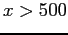, we use the if statement if(grid->xv[ib]>500) to specify these boundary edges. The first part of the if statement sets the flux at the open boundary over all the vertical levels with
      for(k=grid->etop[j];k<grid->Nke[j];k++)
ub[j][k] = -phys->h[ib]*sqrt(prop->grav/(grid->dv[ib]));

which is identical to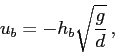and this is the linearized shallow-water free-surface boundary condition (Note that the upwind depth and free-surface height are being used to approximate the values at the open boundary). This loop uses the Nke[j] variable, which is the number of vertical levels at face j, and etop[j], which is the index of the top level at the boundary. The variable etop[j] is usually 0 unless filling and emptying occur at the boundary edges.

In addition to setting the boundary flux in the OpenBoundaryFluxes function, the user must also set the Cartesian components of velocity in the BoundaryVelocities function. These are used to compute the advection of momentum. They can also be used to specify boundary velocities for no-slip type 4 boundary conditions. At the open outflow boundary, the boundary velocity components are set to the upwind value of the velocity component. For the u-component of velocity, for example, the boundary value is specified with

phys->boundary_u[jptr-grid->edgedist][k]=phys->uc[ib][k];

Here, uc is the u-component of velocity at the Voronoi point with index ib.

At the inlet, only the Cartesian velocity components need to be specified. In this example, the u-component of velocity at the inlet is set with the code

phys->boundary_u[jptr-grid->edgedist][k]=
prop->amp*cos(prop->omega*prop->rtime);

and the other components are set to 0. This function uses the variables amp and omega which are set in suntans.dat, and rtime is the physical time in the simulation. The Cartesian velocities which are specified at the inlet boundary are then used to compute the flux in the OpenBoundaryFluxes function with
ub[j][k]=phys->boundary_u[jptr-grid->edgedist][k]*grid->n1[j]+
phys->boundary_v[jptr-grid->edgedist][k]*grid->n2[j];

This is just the dot product of the velocity field specified at the boundary with the normal vector at the boundary edge, which points into the domain by definition. Examples of how to employ velocity boundary conditions are described in Sections 6.5 and 6.7. Section 6.5 also demonstrates how to specify salinity and temperature at the boundaries.Next: Open or stage-specified boundary Up: Specifying boundary conditions Previous: Specifying boundary conditions   Contents
2014-08-06